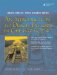# public, protected, and private Derivation

Most of the time, you will see classes using public derivation. For example,

```class Square : public Shape {
// ...
};
```

public derivation describes an interface relationship between two classes. This means that the interface (public part) of the base class merges with the interface of the derived class. When there is an is-a relationship between the derived and base class types, public derivation is appropriate.

Much less commonly you will see protected or private derivation. This is considered an implementation relationship, rather than an is-a relationship. The base class interface (public part) gets merged with the implementation (private or protected, depending on the kind of derivation) of the derived class. In effect, private derivation is like adding an extra object as a private data member to your class.

Similarly, protected derivation is like adding an object as a protected data member to the derived class that shares its this pointer.

Example 23.9 is a concrete example of a situation in which private derivation might be appropriate. The template class Stack is privately derived from QList. The rationale for doing this is that a stack is, by definition, a datastructure that limits access to the top item. The class QStack, which is publicly derived from QVector, has the expected public interface for a stack but it also allows client code unlimited access to the items in the stack because it contains the entire public interface of QVector. Our Stack class is privately derived from QList, so its public interface limits client code access to the handful of stack operations that are consistent with the definition of that data structure.

Example 23.9. src/privatederiv/stack.h

 ```#ifndef _STACK_H_ #define _STACK_H_ #include template class Stack : private QList { public: bool isEmpty() const { return QList::isEmpty(); } T pop() { return takeFirst(); } void push(const T& value) { prepend(value); } const T& top() const { return first(); } int size() const { return QList::size(); } void clear() { QList::clear(); } }; #endif ```

Example 23.10 shows that an attempt by client code to make use of the Stack's base class (QList) interface is not allowed.

Example 23.10. src/privatederiv/stack-test.cpp

 ```#include "stack.h" #include #include using namespace qstd; int main() { Stack strs; strs.push("hic"); strs.push("haec"); strs.push("hoc"); //strs.removeAt(2); <-- 1 int n = strs.size(); cout << n << " items in stack" << endl; for(int i = 0; i < n; ++i) cout << strs.pop() << endl; } ```   (1)Errorinherited QList methods are private.

So, private derivation provides a way to hide the public interface of a base class that was only needed for implementation purposes. What about protected derivation?

Suppose we wish to derive XStack, a particular kind of stack, from the Stack class. With Stack privately derived from QList, we will not be able to make use of any QList member functions when we implement XStack.

If we need to use some of the QList functions when we implement XStack, then we must use protected derivation when we derive Stack from QList.

protected derivation makes the public interface of QList protected in Stack.

Internally, this allows classes derived from Stack to make use of the inherited QList protected interface.An Introduction to Design Patterns in C++ with Qt 4
ISBN: 0131879057
EAN: 2147483647
Year: 2004
Pages: 268